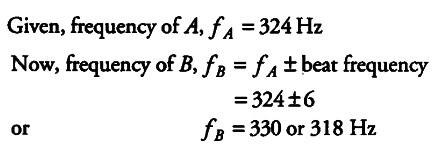# Two sitar strings A and B playing the note 'Ga'

Two sitar strings A and B playing the note ‘Ga’ are slightly out of tune and produce beats of frequency 6 Hz. The tension in the string A is slightly reduced and the beat frequency is found to reduce to 3 Hz. If the original frequency of A is 324 Hz, what is the frequency ofB?Now, if tension in the string is slightly reduced its frequency will also reduce from 324 Hz.
Now, if f_{ B } =330 and f_{ A } reduces, then beat frequency should increase which is not the case but if f_{ B } =318 Hz and f_{ A } decreases the beat frequency should decrease, which is the case and hence f_{ B } =318 Hz.